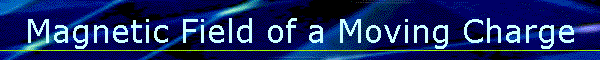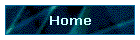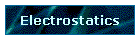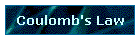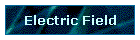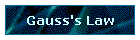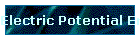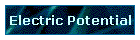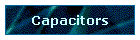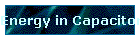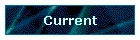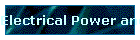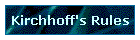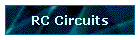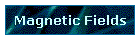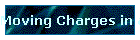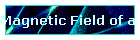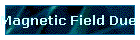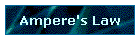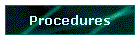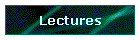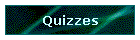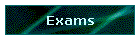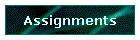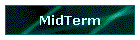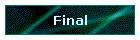Phys 4, Section 2                                                         Feb 20, 2002     Magnetic Field of a Moving Charge Experiments show that the magnetic field of of moving charge can be expressed as: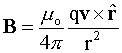μo ≡ 4π × 10-7 N·s2/C2 is called the permeability of free space. The constant εo that is used in electric field calculations is called the permittivity of free space. Note that εoμo = 1/c2. Example: Two protons with a vertical displacement of r between them move in the x-y plane parallel to the x-axis at the same speed v (small compared to c). When they are both at x = 0, what is the ratio of the electric/magnetic forces between them? FE = kq2/r2 To get FB acting on top charge, first find B caused by bottom charge: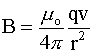So, the force this field exerts on the top charge is: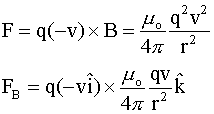Comparing the ratio of FB to FE: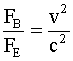Last modified on February 27, 2002